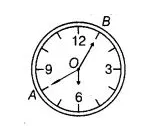# The length of the minute hand of a clock is 5 cm.

Question:

The length of the minute hand of a clock is 5 cm. Find the area swept by the minute hand during the time period 6 : 05 am and 6 : 40 am

Solution:

We know that, in $60 \mathrm{~min}$, minute hand revolving $=360^{\circ}$In 1 min, minute hand revolving $=\frac{360^{\circ}}{60^{\circ}}$

$\therefore \quad$ In $(6: 05$ am to $6: 40 \mathrm{am})=35 \mathrm{~min}$,

minute hand revolving $=\frac{360^{\circ}}{60^{\circ}} \times 35=6 \times 35$

Given that, length of minute hand $(r)=5 \mathrm{~cm}$.

$\therefore$ Area of sector $A O B A$ with angle $\angle O=\frac{\pi r^{2}}{360} \times \angle O$

$=\frac{22}{7} \frac{(5)^{2}}{360^{\circ}} \times 6 \times 35$

$=\frac{22}{7} \times \frac{5 \times 5}{360^{\circ}} \times 6 \times 35$

$=\frac{22 \times 5 \times 5 \times 5}{60^{\circ}}=\frac{22 \times 5 \times 5}{12}$

$=\frac{11 \times 5 \times 5}{6}=\frac{275}{6}=45 \frac{5}{6} \mathrm{~cm}^{2}$

Hence, the required area swept by the minute land is $45 \frac{5}{6} \mathrm{~cm}^{2}$.